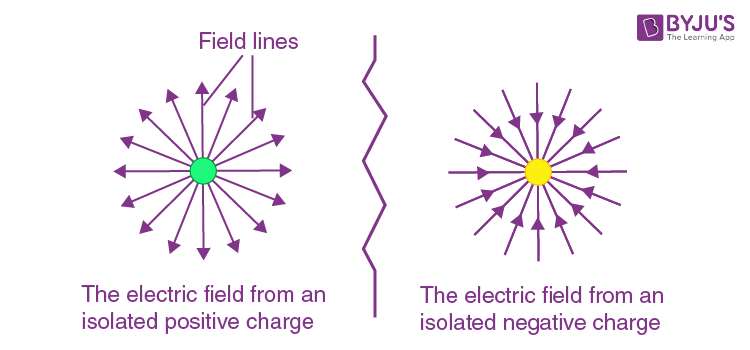# Electric field due to a single charge is ____?

Electric field due to single charge is spherically symmetric.

### Explanation

The electric field is defined as the electric force per unit charge.  The field due to a point charge has spherical symmetry. The electric field is radially outward from the point charge in all directions. The SI unit of the electric field is newton per coulomb which is equal to volts per meter.

• When the electric field is constant at every point, the field is called the uniform electric field. The constant field is obtained by placing the two-conductor parallel to each other, and the potential difference between them remains the same at every point.
• The field which is irregular at every point is called the non-uniform electric field. The non-uniform field has a different magnitude and directions.
• The field is strong when the lines are close together, and it is weak when the field lines move apart from each other.

## Electric field of a point charge

The electric field of a point charge exhibits spherical symmetry since the electric field has the same magnitude on every point of an imaginary sphere centered around the charge q.

The direction of the field is taken as the direction of the force which is exerted on the positive charge. The electric field is radially outwards from positive charge and radially in towards negative point charge.### Articles to Explore:(36)(6)